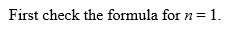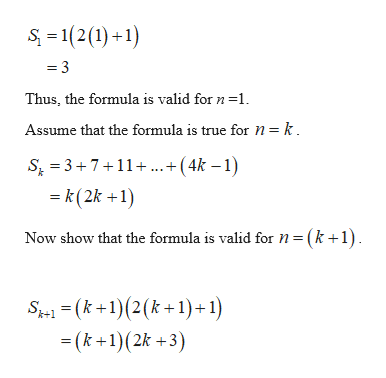# For Exercise, use mathematical induction to prove the given statement for all positive integers n3+7+11+..........+(4n-1)=n(2n+1)

Question
1 views

For Exercise, use mathematical induction to prove the given statement for all positive integers n

3+7+11+..........+(4n-1)=n(2n+1)

check_circle

Step 1

Consider the formula

Step 2...help_outlineImage TranscriptioncloseS = 1(2(1) +1) = 3 Thus, the formula is valid for n =1. Assume that the formula is true for n= k. S, = 3+7+11+...+(4k – 1) = k(2k +1) Now show that the formula is valid for n= (k+1). S = (k +1)(2(k+1) + 1) = (k +1)(2k +3) fullscreen

### Want to see the full answer?

See Solution

#### Want to see this answer and more?

Solutions are written by subject experts who are available 24/7. Questions are typically answered within 1 hour.*

See Solution
*Response times may vary by subject and question.
Tagged in

### Other